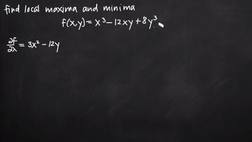PLAY PREVIEW

### Segments in this Video

#### Finding the Local Maxima and Minima of a Function(03:20)

FREE PREVIEW

To find local maxima and minima of a function, use the second derivative test and set the first order partial derivatives equal to 0.

#### Simultaneous Equations(03:34)

A system of simultaneous equations can help simplify the problem.

#### The Second Derivative Test(02:57)

The second derivative test is used to find local maxima and local minima of a multivariable function.

To use the second derivative test to find local maxima and local minima of a multivariable function, you need to find the critical points of the function and determine whether the second order partial derivative with respect to x is positive or negative at each critical point.

#### Credits(00:00)

Credits

For additional digital leasing and purchase options contact a media consultant at 800-257-5126
(press option 3) or sales@films.com.

# Local extrema and saddle points

Part of the Series : Integral Calc: Calculus 3
 3-Year Streaming Price: \$49.95

Share

### Description

This video tutorial works through math problems/equations that address topics in Calculus 3, Partial Derivatives. This specific tutorial addresses Local extrema and saddle points.

Length: 12 minutes

Item#: BVL275721Closed Captioned